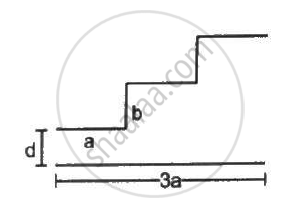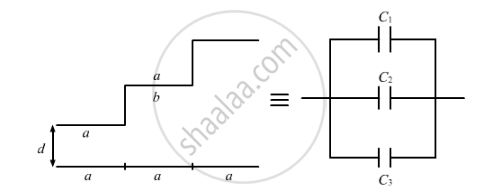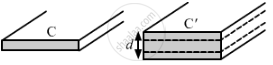Department of Pre-University Education, Karnataka course PUC Karnataka Science Class 12
Share

# A Capacitor is Made of a Flat Plate of Area a and a Second Plate Having a Stair-like Structure as Shown in Figure . the Width of Each Stair is a and the Height is B. - Physics

ConceptCapacitors and Capacitance

#### Question

A capacitor is made of a flat plate of area A and a second plate having a stair-like structure as shown in figure . The width of each stair is a and the height is b. Find the capacitance of the assembly.#### SolutionThe total area of the flat plate is A. The width of each stair is the same. Therefore, the area of the surface of each stair facing the flat plate is the same, that is, A/3 .From the figure, it can be observed that the capacitor assembly is made up of three capacitors. The three capacitors are connected in parallel.

For capacitor C1, the area of the plates is A/3 and the separation between the plates is d.

For capacitor C2, the area of the plates is A/3 and the separation between the plates is (d + b).

For capacitor C3, the area of the plates is A/3 and the separation between the plates is (+ 2b).

Therefore ,

C_1 = (∈_0A)/(3d)

C_2 = (∈_0A)/(3(d+b)

C_3 = (∈_0A)/(3(d+2b)

As the three capacitors are in parallel combination,

C = C_1 + C_2 + C_3

⇒ C = (∈_0A)/(3d) + (∈_0A)/(3(d+b)) + (∈_0A)/(3(d+2b)

⇒ C = (∈_0A)/3 ((3d^2 + 6bd + 2b^2))/(d(d+b)(d+2b))

Is there an error in this question or solution?

#### Video TutorialsVIEW ALL 

Solution A Capacitor is Made of a Flat Plate of Area a and a Second Plate Having a Stair-like Structure as Shown in Figure . the Width of Each Stair is a and the Height is B. Concept: Capacitors and Capacitance.
S